# Array in C

## Array in C Language with Examples

In this article, I am going to discuss the Array in C Language with Examples. Please read our previous articles, where we discussed the Preprocessing Directives in C. As part of this article, you will learn the following pointers in detail.

1. What are Arrays?
2. How to Declare and Initialize an Array in C?
3. How to Access an Array?
4. Why do we need Array?
5. Properties of Array in C
6. Assigning Values to Array in C
7. Runtime Array initialization in C
8. Access Array Elements in C
11. Operations on Array
12. Types of C Arrays
##### What are Arrays in C?

The array is defined as a collection of similar data elements. If you have some sets of integers, and some sets of floats, you can group them under one name as an array.

##### Method of declaring an array

If you want an integer-type array, let’s say int A;

Then, you will get 5 integers, and the array name is “A” and you can access all those integers using the name “A”. So, “A” is an array and you get 5 integers spaces and the indices will be 0,1,2,3,4. So, 5 locations mean indices will start from 0 and end at 4, a total of 5 spaces. For a better understanding, please have a look at the below image.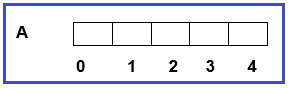Now we can store 5 integers. Every location is an integer. If we assume an integer is taking 2 bytes then there are a total of 10 bytes i.e. 2 bytes each. Now each location can be accessed like A=27; //Here, 27 will store in array “A” with indices 0. Now if I store A=10; //10 is stored in a place with indices 1. For a better understanding, please have a look at the below image,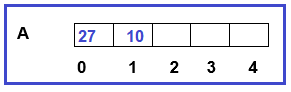So, we can have a group of elements in a single place.

##### How to Declare and Initialize an Array in C Language?

Now I’ll show you how to declare and initialize an array. In the main function, suppose I want to declare an array “A” of size 5. Then you need to declare the array as shown below.

```int main()
{
int A;
}
```

Now, an array of size 5 will be created. When a program is running, then it is running inside the main memory. The main memory is divided into 3 sections i.e. Code section, the Stack section & Heap section as shown in the below image.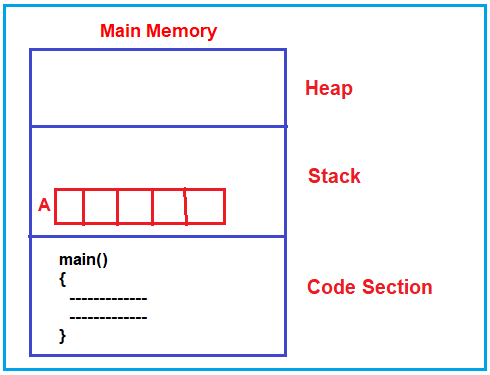As you can see in the above image, whatever code we write will be inside the code section. The point that you need to understand is, the variables that we declared, will be created inside the Stack section. So, here the Array is also going to be created inside the Stack as the array is also a variable.

Those variables that are created inside the stack section are directly accessed by the Main method from the code section. So, the array is directly accessible to the main function and can directly store the values in the array.

Now, I hope you understand how the array is declared and where the array is created inside the main memory.

##### How to declare and initialize an array?

You can declare and initialize an array in the same line as shown in the below example.

```int main()
{
int A={1,3,5,7,9};
}
```

The above code shows the declaration of an array with size 5, along with this, the array is also initialized with the values 1,3,5,7,9. So, this is a declaration as well as the initialization of an array. The memory representation of the above is shown below.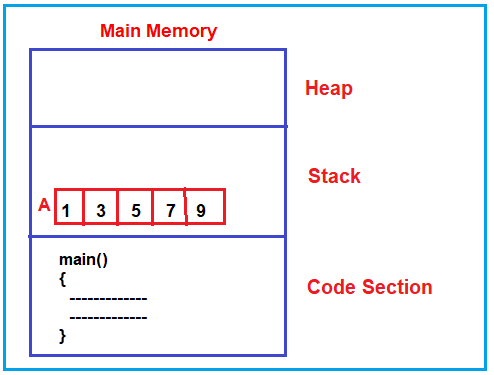##### How to access an array?

We can access all the elements of an array one by one using a “for” loop. To understand this better please have a look at the following code.

```int main()
{
int A={2, 4,6,8,10};
int i;
for(i=0;i<5;i++)
{
printf("%d",A[i]);
}
}
```

I hope you understand the basics of the array. Let us proceed and understand the array in depth.

##### What is an Array in C?

An array is a derived data type in C that is constructed from the fundamental data type of the C programming language. An array is a collection of similar types of data elements in a single entity. In implementation when we require ‘n’ no. of values of the same data type, then recommended creating an array.

When we are working with arrays always memory is constructed in a continuous memory location that’s why possible to access the data randomly. When we are working with arrays all values will share the same name with a unique identification value called ‘index’.

Always array index must be required to start with ‘0’ & end with (size-1). When we are working with arrays, we are required to use an array subscript operator i.e. [ ]. Always array subscript operators require one argument of type unsigned integer constant, whose value is always ‘>0’ only.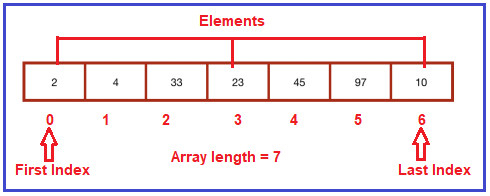##### Why do we need Array?

We can use normal variables (v1, v2, v3, …) when we have a small number of objects, but if we want to store a large number of instances, it becomes difficult to manage them with normal variables. The idea of an array is to represent many instances in one variable. C array is useful if we have to store similar elements.

For example, if we want to store the scores of a student in 6 subjects, then we don’t need to define different variables for the scores in the different subjects. Instead of that, we can define an array that can store the scores in each subject at the contiguous memory locations.

By using the array, we can easily access the elements.

##### Properties of Array in C:

The array contains the following properties:

1. Each element of an array is of the same data type and carries the same size, i.e., int = 4 bytes.
2. Elements of the array are stored at contiguous memory locations where the first element is stored at the smallest memory location.
3. Elements of the array can be randomly accessed since we can calculate the address of each element of the array with the given base address and the size of the data element.
##### Characteristics of Array
1. Arrays are always stored in consecutive memory locations.
2. An array can store multiple values of a similar type which can be referred to by a single name.
3. The array name is actually a pointer to the first location of the memory block allocated to the name of the array.
4. An array either an integer, character or float data type can be initialized only during the declaration but not afterward.
5. Any particular element of an array can be modified separately without distributing other elements.
6. All elements of an array said the same name and they are distinguished from each other with the help of element number.
##### How to declare an array in C?

Syntax: datatype   array_name [ array_size ] ;
Example: take an array of integers ‘n’.
int n;

Here, n[ ] is used to denote an array ‘n’. It means that ‘n’ is an array. So, int n means that ‘n’ is an array of 6 integers. Here, 6 is the size of the array i.e. there are 6 elements in the array ‘n’.We have to specify the array size because the compiler needs to allocate space in the memory which is not possible without knowing the size. The compiler specifies the size required for an array with the help of the number of elements of an array and the size of the data type present in the array.

Here int n‘ will allocate space to 6 integers. We can also declare an array by another method as shown below.

int n[ ] = {2, 3, 15, 8, 48, 13};

In this case, we are declaring and assigning values to the array at the same time. Here, there is no need to specify the array size because the compiler gets it from { 2,3,15,8,48,13 }.

There are various ways in which we can declare an array. It can be done by specifying its type and size, initializing it, or both.

##### Array declaration by specifying size:

int arr1;
With recent C versions, now we can also declare an array of user-specified size
int n = 10;
int arr2[n];

##### Array declaration by initializing elements:

int arr[] = { 10, 20, 30, 40 }
Here, the compiler creates an array of size 4. The above statement is the same as the below statement.
int arr = {10, 20, 30, 40}

##### Array declaration by specifying the size and initializing elements:

int arr = { 10, 20, 30, 40 }
Here, the compiler creates an array of size 6, initializes the first 4 elements as specified by the user, and the rest two elements as 0. The above statement is the same as the below statement.
int arr[] = {10, 20, 30, 40, 0, 0}

##### Index of an Array

In an array, every element has its index and we can access any element by using its index.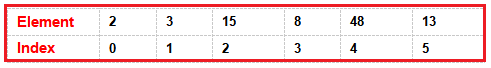0, 1, 2, 3, 4, and 5 are indices. It is like they are the identity of 6 different elements of an array. The index always starts at 0. So, the first element of an array has an index of 0.

Note: The index of an array starts with 0. We access any element of an array using its index:
Syntax: array_name[index]
For example: if the name of an array is ‘n’, then to access the first element (which is at 0 indexes), we write n.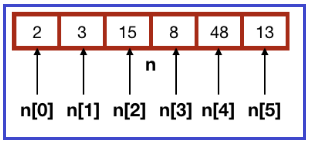Here,
n is 2
n is 3
n is 15
n is 8
n is 48
n is 13

Note: n, n, etc. are like any other variables we were using till now i.e., we can set there value as n = 5; like we do with any other variables (x = 5;, y = 6;, etc.).

##### Assigning Values to Array in C:

By writing int n[ ]={ 2,4,8 };, we are declaring and assigning values to the array at the same time, thus initializing it. But when we declare an array like int n;, we need to assign values to it separately. Because ‘int n;’ will allocate space for 3 integers in memory but there are no integers in that space. To initialize it, assign a value to each of the elements of the array as shown below.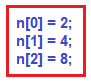It is just like we are declaring some variables and then assigning values to them.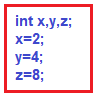Thus, the first way of assigning values to the elements of an array is by doing so at the time of its declaration i.e. int n[ ]={ 2,4,8 }; And the second method is declaring the array first and then assigning values to its elements as shown below.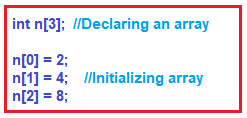You can understand this by treating n, n, and n as similar to the different variables you used before. Just like a variable, an array can be of any other data type also.

float f[ ]= { 1.1, 1.4, 1.5}; Here, ‘f’ is an array of floats.

###### Program: Array Example in C Language
```#include <stdio.h>
int main()
{
int  marks;
float  average;
printf("Enter marks of first student\n");
scanf(" %d" , &marks);
printf("Enter marks of second student\n");
scanf(" %d" , &marks);
printf("Enter marks of third student\n");
scanf(" %d" , &marks);
average = (marks + marks + marks) / 3.0;
printf ("Average marks : %f\n" , average);
return 0;
}```
###### Output: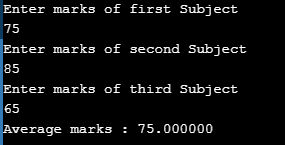In the above example, the average value should be of type ‘float’ because the average of integers can be float also. While taking out the average, the sum of the numbers should be divided by 3.0 and not 3, otherwise, you will get the average value as an integer and not float.

Note: If we have declared an array with some array size and assigned values to only some elements of that array, then the values of other elements are automatically assigned zero.

Suppose we declare and initialize an array as int n = { 12, 13, 5 }; This means that n=12, n=13 and n=5 and rest all elements are zero i.e. n=0 and n=0.

Similarly,

int n;
n = 12;
n = 13;
n = 5;

In the above code, n, n and n are initialized to 12, 13 and 5 respectively. Therefore, n and n are both 0.

Note: Array allocates contiguous memory. Thus, if the address of the first element of an array of integers is 223698688 then the address of the second element will be 223698692 (223698688+4 (4 is the size of one integer)) and the third will be 223698696, and so on. This means that the memories of all elements of an array are allocated together and are continuous.

##### Runtime Array initialization in C:

An array can also be initialized at runtime using scanf() function. This approach is usually used for initializing large arrays or to initialize arrays with user-specified values.

###### Runtime Array initialization Examples in C:
```#include<stdio.h>
void main()
{
int arr;
int i, j;
printf("Enter array element :");
for(i = 0; i < 3; i++)
{
scanf("%d", &arr[i]);    //Run time array initialization
}
printf("Array elements are : ");
for(j = 0; j < 3; j++)
{
printf("%d\n", arr[j]);
}
}```
###### Output: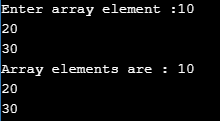##### Input data into the array in C Language

Here, the size of the array is 5. Inside the loop, we are displaying a message to the user to enter the number. All the input values are stored in similar array elements using the scanf function.

for (i=0; i<5; i++)
{
printf(“Enter the number %d \n”, (i+1));
scanf(“%d”, &num[i]);
}

###### Reading out data from an array

If we want to display the elements of the array then we can display like this.
for (i=0; i<5;i++)
{
printf(“num[%d]\n”, num[i]);
}

##### Access Array Elements in C Language:

We can access elements of an array by index. Suppose we declared an array n. The first element is n, the second element is n, and so on.Arrays have 0 as the first index, not 1. For example, n is the first element. If the size of an array is 7, to access the last element, the 7-1 index is used. For example n=10

Note: Array allocates contiguous memory. Thus, if the address of the first element of an array of integers is 223698684 then the address of the second element will be 223698688 (223698684+4 (4 is the size of 1 integer)) and the third will be 223698692, and so on. This means that the memories of all elements of an array are allocated together in a continuous manner.

##### Facts about Array in C:

Array elements are accessed by using an integer index. Array index starts with 0 and goes till the size of the array minus 1.

###### Array Example in C Language:
```#include <stdio.h>
int main()
{
int arr;
arr = 5;
arr = -10;
arr[3 / 2] = 2; // this is same as arr = 2
arr = arr;
printf("%d %d %d %d", arr, arr, arr, arr);
return 0;
}```

Output: 5 2 -10 5

There is no index out of bounds checking in C, for example, the following program compiles fine but may produce unexpected output when run.

```#include <stdio.h>
int main()
{
int arr;
printf("%d ", arr);
printf("%d ", arr[-2]);
return 0;
}```

Output: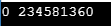In C, it is not a compiler error to initialize an array with more elements than the specified size. For example, the below program compiles fine and shows just a Warning.

```#include <stdio.h>
int main()
{
// Array declaration by initializing it with more elements than specified size.
int arr = { 10, 20, 30, 40, 50 };
return 0;
}```
###### Output: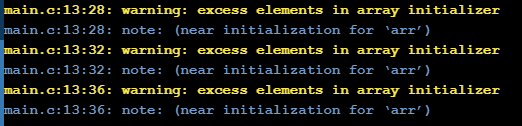The array elements are stored at contiguous memory locations. The following C program demonstrates that array elements are stored in contiguous locations

```#include <stdio.h>
int main()
{
// an array of 10 integers. If arr is stored at address x,
// then arr is stored at x + sizeof(int)
// arr is stored at x + sizeof(int) + sizeof(int) and so on.
int arr, i;
printf("Size of integer in this compiler is %lu\n", sizeof(int));
for (i = 0; i < 5; i++)
// The use of '&' before a variable name, yields address of variable.
printf("Address arr[%d] is %p\n", i, &arr[i]);
return 0;
}```
###### Output: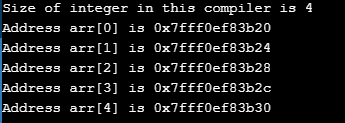##### Advantages of Arrays in C Language
1. Code Optimization: By using an array only a few lines of code are required to access the data.
2. Ease of traversing: By using the for loop, we can easily traverse the elements of an array.
3. Ease of sorting: Only a few lines of code are required to sort the elements of an array.
4. Random Access: We can randomly access any of the elements by using an array.
##### Disadvantage of Arrays in C Language

Fixed Size: Whatever size, we define at the time of declaration of the array, we can’t exceed the limit. So, it doesn’t grow the size dynamically.

Insertion and deletion Costly: Insertion and deletion of elements can be costly since the elements are needed to be managed in accordance with the new memory allocation.

##### Operation of an Array:
1. Traversing: Process each and every element in the array sequentially.
2. Searching: Searching an element to find out whether the element is present or not.
3. Sorting: Arranging the element in an array in a particular sequence.
4. Inserting: To insert the element into the array.
5. Deleting: To delete the element from the array.
##### Types of C Arrays:

There are 2 types of C arrays. They are,

1. One dimensional array
2. Multi-dimensional array (Two-dimensional array, Three-dimensional array, Four-dimensional array, etc…)